# Python Matplotlib drawing contour map

2022-01-31 17:02:12This is my participation 11 The fourth of the yuegengwen challenge 15 God , Check out the activity details ：2021 One last more challenge

# Preface

We were in the past matplotlib.pyplot() Method learning , By now we have been able to draw a line chart 、 Histogram 、 Scatter and other regular charts （ The contents of previous periods are as follows , You can easily view the contents of previous periods ）

stay matplotlib.pyplot In addition to drawing regular charts, such as broken lines 、 Columnar 、 Take it easy and wait , It can also draw the contour map commonly used in geographical plane display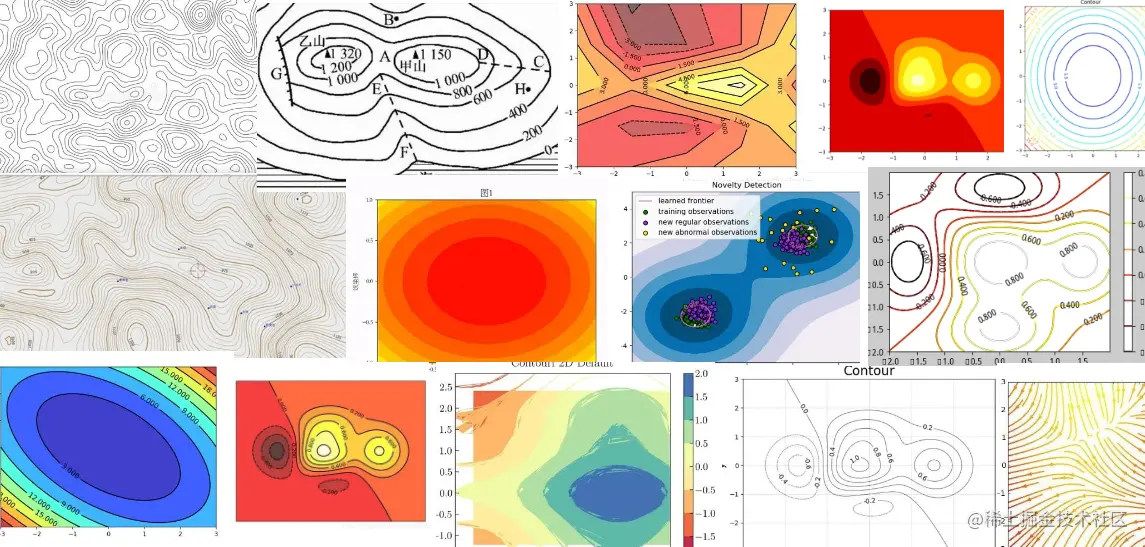In this issue , We will study in detail matplotlib Drawing contour map related attributes of learning ,let's go~

# 1. Contour map Overview

• ## What is a contour map ？

• Contour map is also called horizontal map , adopt 2D Form show 3D Graph of images
• Contour map is also called contour line map , Connect the points with the same surface height into a loop line and display it on the plane curve
• Contour map is also called Z Slice , The dependent variable Z And independent variables X,Y Change and change
• The contour map can be divided into the first curve 、 Meter curve 、 Intermediate curve and auxiliary curve
• ## Contour map common scenes

• Contour maps are often used to show the terrain of a place
• The contour map can also calculate the height of local mountains
• Contour maps are often used in geology 、 Drawn by geographical survey
• Contour maps can also be used to draw circles 、 Mathematical formulas such as ellipse show
• ## To draw a contour map

1. Import matplotlib.pyplot modular
2. Prepare the data , have access to numpy/pandas Collating data
3. call pyplot.contour() perhaps pyplot.contourf() Draw contours
• ## The case shows

Contour map drawing needs the help of many trigonometric functions learned in high school 、 Exponential function and other formulas , In this case, we use the contour method to summarize circles

• Case data preparation

• np.arrage() Prepare a series of continuous data
• np.meshgrid() Convert data into a matrix
``````import numpy as np
#  Define a continuous set of data

x_value = np.arange(-5,5,0.1)
y_value = np.arange(-5,5,0.1)

#  Convert to matrix data
x,y = np.meshgrid(x_value,y_value)
Copy code ``````
• Draw contours

``````
import matplotlib.pyplot as plt

plt.contour(x,y,z)

plt.title("Display Contour")
plt.xlabel("x(m)")
plt.ylabel("y(m)")

plt.show()

plt.show()
Copy code ``````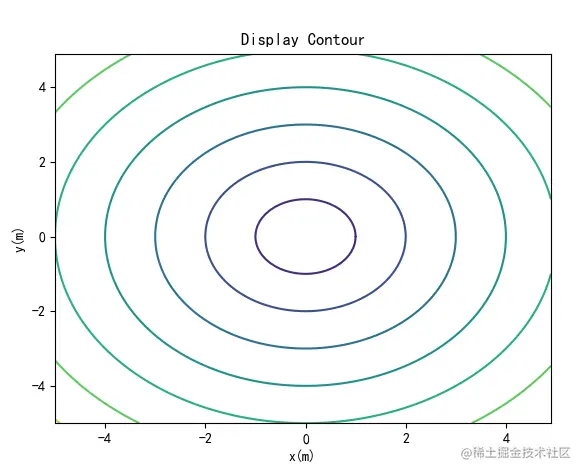# 2. Contour map properties

• ## Set the contour color

• keyword ：colors
• Value range :
• English words for color ： Like red "red"
• Abbreviations of words indicating color, such as ： Red "r", yellow "y"
• RGB Format ： Hexadecimal format, such as "#88c999";(r,g,b) Tuple form
• You can also pass in a color list
• ## Set contour transparency ：

• keyword ：alpha
• The default is 1
• The value range is ：0~1
• ## Sets the contour color level

• keyword ：cmap
• colors and cmap Two keywords cannot be provided at the same time
• The value is ： The registered color indicates
• In the form of ：" color table _r"
• Commonly used ：'Accent', 'Accent_r', 'Blues', 'Blues_r', 'BrBG', 'BrBG_r', 'BuGn', 'BuGn_r', 'BuPu', 'BuPu_r', 'CMRmap', 'CMRmap_r', 'Dark2', 'Dark2_r', 'GnBu', 'GnBu_r', 'Greens'
• ## Sets the contour width

• keyword ：linewidths
• The default contour width is 1.5
• The value can be float Type or list
• ## Set the contour style

• keyword ：linestyles
• The default value is ：solid
• The value is optional ：{None, 'solid', 'dashed', 'dashdot', 'dotted'}
• linestyles by None When the line is monochrome , The lines with negative contour will be set to dashed
• We added some attributes to the contour map in the previous section

• The line is red , The line width gradually increases , The line style is dashed, Transparency set to 0.5

`````````python
plt.contour(x,y,z,colors="r",
linestyles="dashed",
linewidths=np.arange(0.5,4,0.5),alpha=0.5)
```
Copy code ``````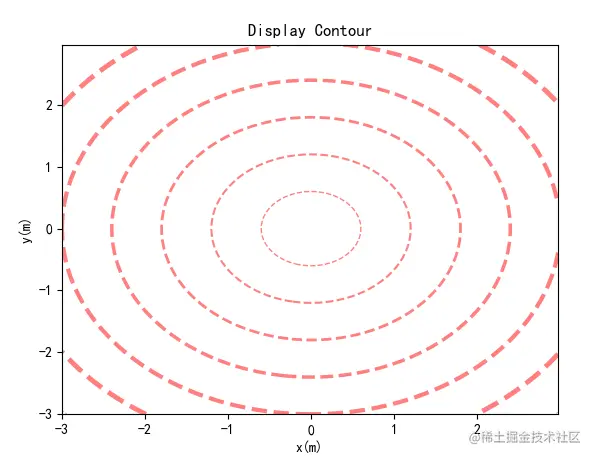• Pass in colors list

``````plt.contour(x,y,z,
colors=('r','green','blue',(1,1,0),"#afeeee","0.5"),
linewidths=np.arange(0.5,4,0.5))
Copy code ``````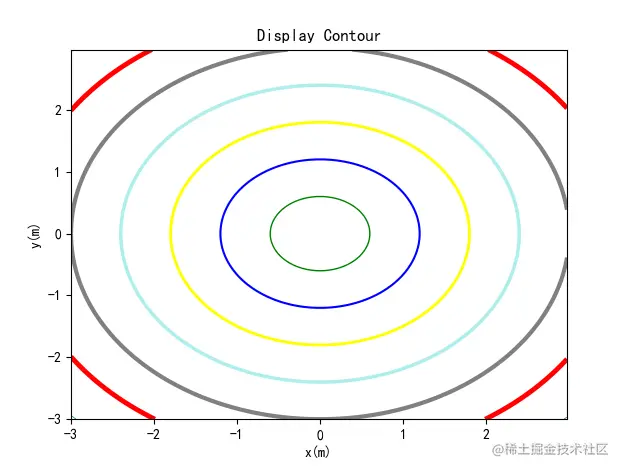• Is a contour map , Set up cmap It's red

``````z = np.exp(-x**2-y**2)
z1 = np.exp(-(x-1)**2-(y-1)**2)
Z = (z-z1)*2

plt.contour(x,y,Z,
cmap='afmhot_r',
linewidths=np.arange(0.5,4,0.5))
Copy code ``````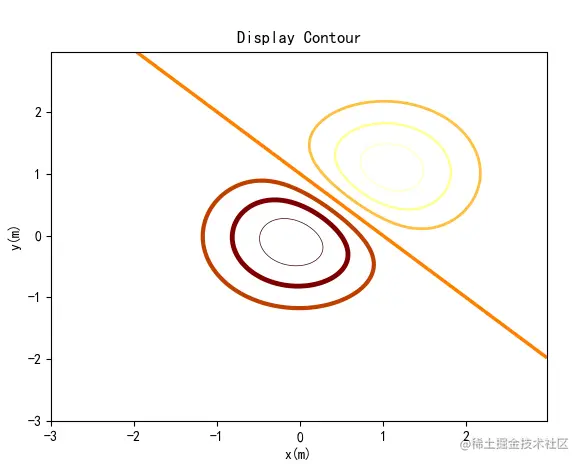# 3. Show outline labels

When we look at the contour map , The outline tab will help us better view the chart . Add outline labels , We need the help of clabe

• pyplot.contour() Method of drawing contour lines , Returns the QuadContourset

• QuadContourset contain level The list of data

• Use pyplot.clabel() Accept level List data is marked on contour lines

``````
x_value = np.arange(-3,3,0.025)
y_value = np.arange(-3,3,0.025)

x,y = np.meshgrid(x_value,y_value)

z = (1-x**2+y**5)*np.exp(-x**2-y**2)

cs = plt.contour(x,y,z,cmap="Blues_r",linewidths=np.arange(0.5,4,0.5))

plt.clabel(cs,fontsize=9,inline=True)
Copy code ``````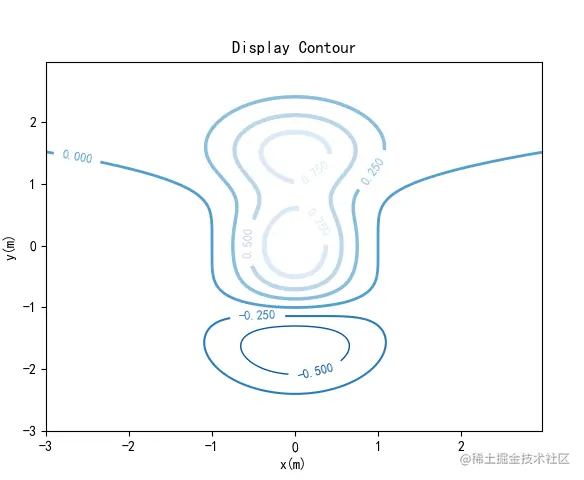# 4. Fill color

Usually in contour plots , Different areas are filled with different colors , Help us better understand when looking at the chart

• Use pyplot.contourf() Contrast the contour of the same area for filling color

``````z = (1-x**2+y**5)*np.exp(-x**2-y**2)

cs = plt.contour(x,y,z,10,colors="b",linewidths=0.5)

plt.clabel(cs,fontsize=12,inline=True)

plt.contourf(x,y,z,10,cmap="Blues_r",alpha=0.75)
Copy code ``````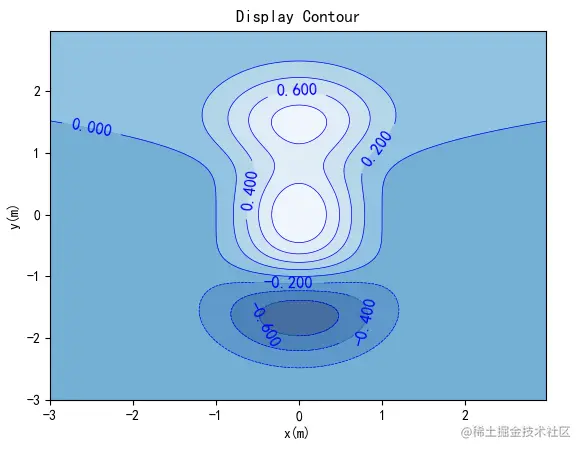# 5. Add color bar description

We can use pyplot.colorbar() Method to add a color bar description

``````z = (x**2+y**5)*np.exp(-x**2-y**2)
z1 = np.exp(-(x-1)**2-(y-1)**2)
Z = (z-z1)*2

cs = plt.contour(x,y,Z,10,colors="black",linewidths=0.5)

plt.clabel(cs,fontsize=12,inline=True)

plt.contourf(x,y,Z,10,cmap="afmhot_r",alpha=0.5)

plt.colorbar(shrink=0.8)
Copy code ``````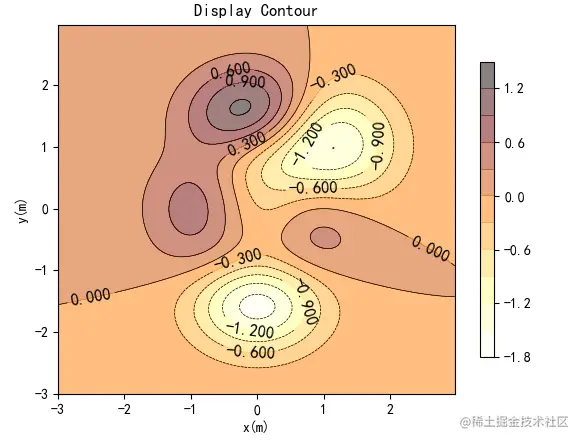# summary

In this issue , Yes matplotlib.pyplot Method of drawing contour lines contour and contourf Learning related attributes . When drawing contour maps , We need to do trigonometric functions 、 Exponential function 、 Have a little knowledge of sine and cosine functions , To draw the chart you want

During the study of this section , High school math knowledge is returned to the teacher , Feel the head , Why did you lose your hair again

The above is the content of this issue , Welcome big guys to praise and comment , See you next time ~